NCERT Solutions: Circles (Exercise 9.2)

# Circles (Exercise 9.2) NCERT Solutions - Mathematics (Maths) Class 9

Q1. Two circles of radii 5 cm and 3 cm intersect at two points and the distance between their centres is 4 cm. Find the length of the common chord.
Ans: Given parameters are:
OP = 5cm
OS = 4cm and
PS = 3cm
Also, PQ = 2PR
Now, suppose RS = x. The diagram for the same is shown below.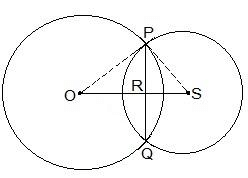Consider the ΔPOR,
OP2 = OR2 + PR2
⇒ 52 = (4 - x)2 + PR2
⇒ 25 = 16 + x2 - 8x + PR2
∴ PR2 = 9 - x2 + 8x — (i)
Now consider ΔPRS,
PS2 = PR2 + RS2
⇒ 32 = PR2 + x2
∴ PR2 = 9 - x2 — (ii)
By equating equation (i) and equation (ii) we get,
9 - x2 + 8x = 9 - x2
⇒ 8x = 0
⇒ x = 0
Now, put the value of x in equation (i)
PR2 = 9 - 02
⇒ PR = 3cm
∴ The length of the cord i.e. PQ = 2PR
So, PQ = 2 × 3 = 6cm.

Q2. If two equal chords of a circle intersect within the circle, prove that the segments of one chord are equal to corresponding segments of the other chord.
Ans:
Let AB and CD be two equal cords (i.e. AB = CD). In the above question, it is given that AB and CD intersect at a point, say, E.
It is now to be proven that the line segments AE = DE and CE = BE
Construction Steps:
Step 1: From the center of the circle, draw a perpendicular to AB i.e. OM ⊥ AB
Step 2: Similarly, draw ON ⊥ CD.
Step 3: Join OE. Now, the diagram is as follows-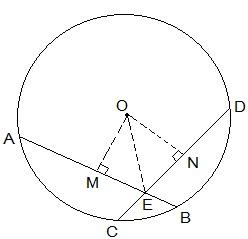Proof:
From the diagram, it is seen that OM bisects AB and so, OM ⊥ AB
Similarly, ON bisects CD and so, ON ⊥ CD
It is known that AB = CD. So,
AM = ND — (i)
and MB = CN — (ii)
Now, triangles ΔOME and ΔONE are similar by RHS congruency since
OME = ONE (They are perpendiculars)
OE = OE (It is the common side)
OM = ON (AB and CD are equal and so, they are equidistant from the centre)
∴ ΔOME ΔONE
ME = EN (by CPCT) — (iii)
Now, from equations (i) and (ii) we get,
AM + ME = ND + EN
So, AE = ED
Now from equations (ii) and (iii) we get,
MB - ME = CN - EN
So, EB = CE (Hence proved).

Q3. If two equal chords of a circle intersect within the circle, prove that the line joining the point of intersection to the centre makes equal angles with the chords.
Ans: From the question we know the following:
(i) AB and CD are 2 chords which are intersecting at point E.
(ii) PQ is the diameter of the circle.
(iii) AB = CD.
Now, we will have to prove that BEQ = CEQ
For this, the following construction has to be done:
Construction:
Draw two perpendiculars are drawn as OM ⊥ AB and ON ⊥ D. Now, join OE. The constructed diagram will look as follows: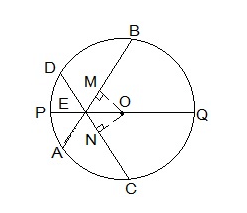Now, consider the triangles ΔOEM and ΔOEN.
Here,
(i) OM = ON [Since the equal chords are always equidistant from the centre]
(ii) OE = OE [It is the common side]
(iii) OME = ONE [These are the perpendiculars]
So, by RHS congruency criterion, ΔOEM ΔOEN.
Hence, by CPCT rule, MEO = NEO
∴ BEQ = CEQ (Hence proved).

Q4. If a line intersects two concentric circles (circles with the same centre) with centre O at A, B, C and D, prove that AB = CD (see Fig).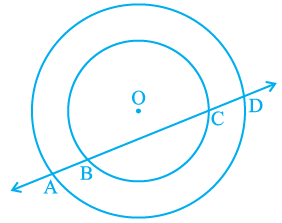Ans: The given image is as follows: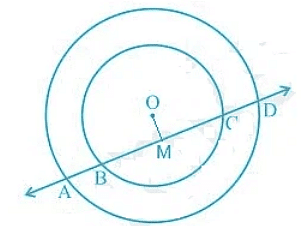First, draw a line segment from O to AD such that OM ⊥ AD.
Therefore, AM = MD — (i)
Also, since OM ⊥ BC, OM bisects BC.
Therefore, BM = MC — (ii)
From equation (i) and equation (ii),
AM - BM = MD - MC
∴ AB = CD.

Q5. Three girls Reshma, Salma and Mandip are playing a game by standing on a circle of radius 5m drawn in a park. Reshma throws a ball to Salma, Salma to Mandip, Mandip to Reshma. If the distance between Reshma and Salma and between Salma and Mandip is 6m each, what is the distance between Reshma and Mandip?
Ans: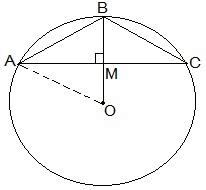Let the positions of Reshma, Salma and Mandip be represented as A, B and C respectively.
From the question, we know that AB = BC = 6cm.
So, the radius of the circle i.e. OA = 5cm
Now, draw a perpendicular BM ⊥ AC.
Since AB = BC, ABC can be considered as an isosceles triangle. M is mid-point of AC. BM is the perpendicular bisector of AC and thus it passes through the centre of the circle.
Now,
let AM = y and
OM = x
So, BM will be = (5 - x).
By applying Pythagorean theorem in ΔOAM we get,
OA2 = OM2 +AM2
⇒ 52 = x2 +y2 — (i)
Again, by applying Pythagorean theorem in ΔAMB,
AB2 = BM2 +AM2
⇒ 62 = (5 - x)2 + y2 — (ii)
Subtracting equation (i) from equation (ii), we get
36 - 25 = (5 - x)2 + y2 - x2 - y2
Now, solving this equation we get the value of x as
x = 7 / 5
Substituting the value of x in equation (i), we get
y2 + (49 / 25) = 25
⇒ y2 = 25 – (49 / 25)
Solving it we get the value of y as
y = 24 / 5
Thus,
AC = 2 × AM
= 2 × y
= 2 × (24 / 5) m
AC = 9.6 m
So, the distance between Reshma and Mandip is 9.6 m.

Q6. A circular park of radius 20m is situated in a colony. Three boys Ankur, Syed and David are sitting at equal distance on its boundary each having a toy telephone in his hands to talk each other. Find the length of the string of each phone.
Ans: First, draw a diagram according to the given statements. The diagram will look as follows.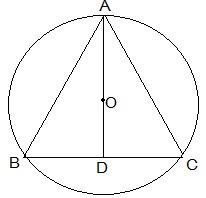Here the positions of Ankur, Syed and David are represented as A, B and C respectively. Since they are sitting at equal distances, the triangle ABC will form an equilateral triangle.
AD ⊥ BC is drawn. Now, AD is median of ΔABC and it passes through the centre O.
Also, O is the centroid of the ΔABC. OA is the radius of the triangle.
OA = 2 / 3 AD
Let the side of a triangle a metres then BD = a / 2 m.
Applying Pythagoras theorem in ΔABD,
⇒ AD2 = AB2 - BD2
⇒ AD2 = a2 - (a / 2)2
⇒ AD2 = 3a2 / 4
⇒ AD = √3a / 2
OA = 2 / 3 AD
20 m = 2 / 3 × √3a / 2
a = 20√3 m
So, the length of the string of the toy is 20√3 m.

The document Circles (Exercise 9.2) NCERT Solutions | Mathematics (Maths) Class 9 is a part of the Class 9 Course Mathematics (Maths) Class 9.
All you need of Class 9 at this link: Class 9

## Mathematics (Maths) Class 9

42 videos|378 docs|65 tests

## FAQs on Circles (Exercise 9.2) NCERT Solutions - Mathematics (Maths) Class 9

 1. What is the formula to find the circumference of a circle?Ans. The formula to find the circumference of a circle is C = 2πr, where C represents the circumference and r represents the radius of the circle.
 2. How is the area of a circle calculated?Ans. The area of a circle is calculated using the formula A = πr^2, where A represents the area and r represents the radius of the circle.
 3. What is the relationship between the radius and diameter of a circle?Ans. The diameter of a circle is twice the length of its radius. In other words, the radius is half the length of the diameter. Mathematically, we can express this relationship as d = 2r, where d represents the diameter and r represents the radius.
 4. Can we find the circumference of a circle if we only know its diameter?Ans. Yes, we can find the circumference of a circle if we only know its diameter. The formula to find the circumference using the diameter is C = πd, where C represents the circumference and d represents the diameter of the circle.
 5. How can we find the radius of a circle if we only know its circumference?Ans. To find the radius of a circle if we only know its circumference, we can use the formula r = C/(2π), where r represents the radius and C represents the circumference of the circle.

## Mathematics (Maths) Class 9

42 videos|378 docs|65 testsExplore Courses for Class 9 examSignup to see your scores go up within 7 days! Learn & Practice with 1000+ FREE Notes, Videos & Tests.
10M+ students study on EduRev
Track your progress, build streaks, highlight & save important lessons and more!
Related Searches

,

,

,

,

,

,

,

,

,

,

,

,

,

,

,

,

,

,

,

,

,

;